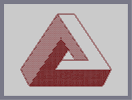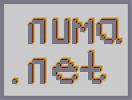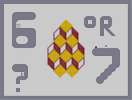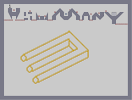### OpTiCaL iLlUsIoN 4Hover over the thumbnail for a full-size version.

Author schmidty563 author:schmidty563 n-art rated 2005-12-28 4 by 59 people. \$OpTiCaL iLlUsIoN 4#schmidty563#none#00000000000000000000000000000000000000000000000000000000000000000000000000000000000000000000000000000000000000000000000000000000000000000000000000000000000000000000000000000000000000000000000000000000000000000000000000000000000000000000000000000000000000000000000000000000000000000000000000000000000000000000000000000000000000000000000000000000000000000000000000000000000000000000000000000000000000000000000000000000000000000000000000000000000000000000000000000000000000000000000000000000000000000000000000000000000000000000000000000000000000000000000000000000000000000000000000000000000000000000000000000000000000000000000000000000000000000000000000000000000000000000000000000000000000000000000000000000000000000|12^348,84!12^360,84!12^372,84!12^384,84!12^396,84!12^408,84!12^420,84!12^432,84!12^444,84!12^354,84!12^366,84!12^390,84!12^402,84!12^414,84!12^426,84!12^438,84!12^180,516!12^192,516!12^204,516!12^216,516!12^228,516!12^240,516!12^252,516!12^264,516!12^276,516!12^288,516!12^300,516!12^324,516!12^312,516!12^336,516!12^348,516!12^360,516!12^372,516!12^384,516!12^396,516!12^408,516!12^420,516!12^432,516!12^444,516!12^456,516!12^468,516!12^480,516!12^492,516!12^504,516!12^516,516!12^528,516!12^540,516!12^552,516!12^564,516!12^576,516!12^588,516!12^600,516!12^612,516!12^186,516!12^198,516!12^210,516!12^222,516!12^234,516!12^246,516!12^258,516!12^270,516!12^282,516!12^294,516!12^306,516!12^318,516!12^330,516!12^342,516!12^354,516!12^366,516!12^378,516!12^390,516!12^402,516!12^414,516!12^426,516!12^438,516!12^450,516!12^462,516!12^474,516!12^486,516!12^498,516!12^510,516!12^522,516!12^534,516!12^546,516!12^558,516!12^570,516!12^582,516!12^594,516!12^606,516!12^618,510!12^624,498!12^630,486!12^636,474!12^642,462!12^648,450!12^660,426!12^450,90!12^456,96!12^456,102!12^462,108!12^468,114!12^468,120!12^474,126!12^480,132!12^480,138!12^486,144!12^492,150!12^492,156!12^498,162!12^504,168!12^504,174!12^510,180!12^516,192!12^516,186!12^522,198!12^528,204!12^528,210!12^534,216!12^540,222!12^540,228!12^546,234!12^552,240!12^552,246!12^558,252!12^564,258!12^564,264!12^570,270!12^576,276!12^576,282!12^582,288!12^588,294!12^588,300!12^594,306!12^600,312!12^600,318!12^606,324!12^612,330!12^612,336!12^618,342!12^624,348!12^624,354!12^630,360!12^636,366!12^636,372!12^642,378!12^648,384!12^648,390!12^654,396!12^660,402!12^660,408!12^666,414!12^666,420!12^660,432!12^654,438!12^654,444!12^648,456!12^642,468!12^636,480!12^630,492!12^624,504!12^174,510!12^168,504!12^168,498!12^162,492!12^162,486!12^156,480!12^156,474!12^150,468!12^150,462!12^144,456!12^144,450!12^138,444!12^138,438!12^132,432!12^132,426!12^126,420!12^126,414!12^132,408!12^138,396!12^144,390!12^144,384!12^150,378!12^156,372!12^156,366!12^162,360!12^168,354!12^168,348!12^174,342!12^180,336!12^180,330!12^186,324!12^192,318!12^192,312!12^198,306!12^204,300!12^204,294!12^210,288!12^216,282!12^216,276!12^222,270!12^228,264!12^228,258!12^234,252!12^240,246!12^240,240!12^246,234!12^252,228!12^252,222!12^258,216!12^264,210!12^264,204!12^270,198!12^276,192!12^276,186!12^282,180!12^288,174!12^288,168!12^294,162!12^300,156!12^300,150!12^306,144!12^312,138!12^312,132!12^318,126!12^324,120!12^324,114!12^330,108!12^336,102!12^336,96!12^342,90!12^348,90!12^354,96!12^354,102!12^360,108!12^366,114!12^366,120!12^372,126!12^378,132!12^378,138!12^384,144!12^390,150!12^390,156!12^396,162!12^402,168!12^402,174!12^408,180!12^414,186!12^414,192!12^420,198!12^426,204!12^426,210!12^432,216!12^438,222!12^438,228!12^444,234!12^450,240!12^450,246!12^456,252!12^462,258!12^462,264!12^468,270!12^474,276!12^474,282!12^480,288!12^486,294!12^486,300!12^492,306!12^498,312!12^498,318!12^504,324!12^510,330!12^510,336!12^660,420!12^654,420!12^642,420!12^648,420!12^636,420!12^630,420!12^624,420!12^618,420!12^606,420!12^612,420!12^600,420!12^594,420!12^588,420!12^582,420!12^576,420!12^570,420!12^564,420!12^558,420!12^552,420!12^546,420!12^540,420!12^534,420!12^528,420!12^516,420!12^522,420!12^510,420!12^504,420!12^492,420!12^498,420!12^486,420!12^480,420!12^474,420!12^468,420!12^462,420!12^456,420!12^450,420!12^444,420!12^438,420!12^432,420!12^426,420!12^420,420!12^414,420!12^408,420!12^402,420!12^396,420!12^390,420!12^384,420!12^378,420!12^372,420!12^240,420!12^180,510!12^186,504!12^186,498!12^192,492!12^198,486!12^198,480!12^204,474!12^210,468!12^210,462!12^216,456!12^222,450!12^222,444!12^228,438!12^234,432!12^234,426!12^246,408!12^252,402!12^258,396!12^258,390!12^264,384!12^270,378!12^270,372!12^276,366!12^282,360!12^282,354!12^288,348!12^294,342!12^294,336!12^300,330!12^306,324!12^306,318!12^312,312!12^318,306!12^318,300!12^324,294!12^330,288!12^330,282!12^336,276!12^342,264!12^240,414!12^342,270!12^366,420!12^360,420!12^354,420!12^348,420!12^342,420!12^336,420!12^330,420!12^330,414!12^336,408!12^342,402!12^342,396!12^348,390!12^354,384!12^354,378!12^360,372!12^366,366!12^366,360!12^372,354!12^348,258!12^354,252!12^354,246!12^360,240!12^366,234!12^366,228!12^378,348!12^378,342!12^384,336!12^390,330!12^390,324!12^516,342!12^522,348!12^522,354!12^528,360!12^522,360!12^516,360!12^510,360!12^504,360!12^498,360!12^492,360!12^486,360!12^480,360!12^474,360!12^468,360!12^462,360!12^456,360!12^450,360!12^444,360!12^438,360!12^432,360!12^426,360!12^420,360!12^414,360!12^408,360!12^396,360!12^402,360!12^390,360!12^384,360!12^378,360!12^372,360!12^372,240!12^378,246!12^378,252!12^384,258!12^390,264!12^390,270!12^396,276!12^402,282!12^402,288!12^408,294!12^414,300!12^414,306!12^420,312!12^426,318!12^426,324!12^432,330!12^438,336!12^438,342!12^444,348!12^450,354!12^396,318!12^402,312!12^402,306!12^408,300!12^132,402!12^378,84!12^366,240!12^360,246!12^366,246!12^372,246!12^372,252!12^366,252!12^360,252!12^354,258!12^360,258!12^366,258!12^372,258!12^378,258!12^384,264!12^378,264!12^372,264!12^366,264!12^360,264!12^354,264!12^348,264!12^348,270!12^354,270!12^360,270!12^366,270!12^372,270!12^378,270!12^384,270!12^390,276!12^384,276!12^378,276!12^372,276!12^366,276!12^360,276!12^354,276!12^348,276!12^342,276!12^336,282!12^342,282!12^348,282!12^354,282!12^366,282!12^360,282!12^372,282!12^378,282!12^384,282!12^390,282!12^396,282!12^336,288!12^342,288!12^348,288!12^354,288!12^360,288!12^360,288!12^366,288!12^372,288!12^378,288!12^390,288!12^384,288!12^396,288!12^330,294!12^336,294!12^342,294!12^348,294!12^354,294!12^360,294!12^366,294!12^372,294!12^378,294!12^384,294!12^390,294!12^396,294!12^402,294!12^324,300!12^330,300!12^336,300!12^342,300!12^348,300!12^354,300!12^360,300!12^366,300!12^372,300!12^378,300!12^384,300!12^390,300!12^396,300!12^402,300!12^324,306!12^330,306!12^336,306!12^342,306!12^348,306!12^354,306!12^360,306!12^366,306!12^378,306!12^372,306!12^384,306!12^390,306!12^396,306!12^318,312!12^324,312!12^330,312!12^336,312!12^342,312!12^348,312!12^354,312!12^360,312!12^366,312!12^372,312!12^378,312!12^384,312!12^384,312!12^390,312!12^396,312!12^312,318!12^312,324!12^318,324!12^318,318!12^324,318!12^324,324!12^336,324!12^330,318!12^330,324!12^336,318!12^342,318!12^342,324!12^348,324!12^354,318!12^354,318!12^348,318!12^354,324!12^360,324!12^360,318!12^366,318!12^366,324!12^372,324!12^372,318!12^378,318!12^378,324!12^384,324!12^384,318!12^390,318!12^306,330!12^312,330!12^318,330!12^324,330!12^330,330!12^336,330!12^342,330!12^348,330!12^354,330!12^360,330!12^366,330!12^372,330!12^378,330!12^384,330!12^300,336!12^300,342!12^312,342!12^306,336!12^306,336!12^306,342!12^312,336!12^318,336!12^318,342!12^324,342!12^324,336!12^330,336!12^330,342!12^336,342!12^336,336!12^342,336!12^342,342!12^348,342!12^348,336!12^354,336!12^354,342!12^360,342!12^360,336!12^366,336!12^366,342!12^372,342!12^372,336!12^378,336!12^294,348!12^300,348!12^306,348!12^312,348!12^318,348!12^324,348!12^330,348!12^336,348!12^342,348!12^348,348!12^354,348!12^360,348!12^366,348!12^372,348!12^324,420!12^246,420!12^252,420!12^258,420!12^264,420!12^270,420!12^276,420!12^288,420!12^282,420!12^294,420!12^300,420!12^306,420!12^312,420!12^318,420!12^246,414!12^252,414!12^258,414!12^264,414!12^270,414!12^276,414!12^282,414!12^288,414!12^294,414!12^306,414!12^300,414!12^312,414!12^318,414!12^324,414!12^252,408!12^258,408!12^264,408!12^270,408!12^276,408!12^282,408!12^288,408!12^294,408!12^306,408!12^300,408!12^312,408!12^318,408!12^324,408!12^330,408!12^288,354!12^288,360!12^288,366!12^282,366!12^282,366!12^282,372!12^276,372!12^276,378!12^276,384!12^270,384!12^270,390!12^264,390!12^264,396!12^258,402!12^264,402!12^270,396!12^270,402!12^276,402!12^276,396!12^276,390!12^294,354!12^294,360!12^294,366!12^294,372!12^294,378!12^294,390!12^294,384!12^294,396!12^294,402!12^288,402!12^282,402!12^282,390!12^282,396!12^288,396!12^288,390!12^288,384!12^282,384!12^282,378!12^288,378!12^288,372!12^336,402!12^336,396!12^336,390!12^336,384!12^336,378!12^336,366!12^336,372!12^336,360!12^336,354!12^342,354!12^342,354!12^348,354!12^354,354!12^360,354!12^366,354!12^342,360!12^348,360!12^354,360!12^360,360!12^342,366!12^348,366!12^348,366!12^360,366!12^354,366!12^342,372!12^354,372!12^348,372!12^342,384!12^342,378!12^348,378!12^348,384!12^342,390!12^300,354!12^306,354!12^312,354!12^318,354!12^330,354!12^324,354!12^330,360!12^330,366!12^330,372!12^330,378!12^330,384!12^330,390!12^330,402!12^330,396!12^324,402!12^312,402!12^300,402!12^306,402!12^318,402!12^306,396!12^300,390!12^300,360!12^300,360!12^312,360!12^306,360!12^318,360!12^324,360!12^324,372!12^324,366!12^324,378!12^324,378!12^324,384!12^324,390!12^324,396!12^318,396!12^312,396!12^300,396!12^306,390!12^318,390!12^312,390!12^300,366!12^300,366!12^300,372!12^300,378!12^300,384!12^306,384!12^306,378!12^306,366!12^306,372!12^312,366!12^312,366!12^318,366!12^318,366!12^318,372!12^318,378!12^312,384!12^312,378!12^312,372!12^318,378!12^318,384!12^294,432!12^300,438!12^306,444!12^312,450!12^318,456!12^324,462!12^330,468!12^342,474!12^336,474!12^342,468!12^348,462!12^348,456!12^354,462!12^360,468!12^366,474!12^372,468!12^372,462!12^378,456!12^384,450!12^390,438!12^390,444!12^408,450!12^390,456!12^402,450!12^396,456!12^396,462!12^396,468!12^396,468!12^408,474!12^420,480!12^426,468!12^408,456!12^420,450!12^426,456!12^426,462!12^414,450!12^396,474!12^402,474!12^408,474!12^414,474!12^426,474!12^438,456!12^444,456!12^444,462!12^450,468!12^456,474!12^462,474!12^462,468!12^468,468!12^474,468!12^480,474!12^486,468!12^492,462!12^498,456!12^504,456!12^504,444!12^504,450!12^240,426!12^240,432!12^240,438!12^240,444!12^240,450!12^240,456!12^240,462!12^240,468!12^240,474!12^240,480!12^240,486!12^240,492!12^240,498!12^240,504!12^240,510!12^186,510!12^192,510!12^198,510!12^204,510!12^210,510!12^222,510!12^216,510!12^228,510!12^234,510!12^234,504!12^234,498!12^234,486!12^234,492!12^234,480!12^234,474!12^234,468!12^234,462!12^234,450!12^234,456!12^234,444!12^234,438!12^228,444!12^228,450!12^228,456!12^222,456!12^222,462!12^222,462!12^228,462!12^216,462!12^216,468!12^222,468!12^228,468!12^228,474!12^222,474!12^216,474!12^216,474!12^210,474!12^204,480!12^210,480!12^216,480!12^222,480!12^234,480!12^228,480!12^228,486!12^216,486!12^216,486!12^204,486!12^210,486!12^222,486!12^228,492!12^216,492!12^222,492!12^198,492!12^204,492!12^210,492!12^192,498!12^198,498!12^204,498!12^216,498!12^210,498!12^222,498!12^228,498!12^192,504!12^198,504!12^204,504!12^210,504!12^216,504!12^222,504!12^228,504!12^606,426!12^606,432!12^606,438!12^606,444!12^606,450!12^606,456!12^606,462!12^606,468!12^606,474!12^606,480!12^606,486!12^606,492!12^606,498!12^606,504!12^606,504!12^606,510!12^612,510!12^612,504!12^618,504!12^618,498!12^612,498!12^612,492!12^612,486!12^612,480!12^612,474!12^612,468!12^612,462!12^612,450!12^612,456!12^612,444!12^612,432!12^612,438!12^612,426!12^618,426!12^618,432!12^618,438!12^618,444!12^618,450!12^618,456!12^618,462!12^618,468!12^618,474!12^618,486!12^618,480!12^618,492!12^624,492!12^624,486!12^624,474!12^624,480!12^624,468!12^624,456!12^624,462!12^624,450!12^624,444!12^624,438!12^624,432!12^624,426!12^630,426!12^630,432!12^630,438!12^630,444!12^630,450!12^630,456!12^630,468!12^630,462!12^630,474!12^630,480!12^636,462!12^636,468!12^636,450!12^636,456!12^642,456!12^642,444!12^642,444!12^642,450!12^636,444!12^636,438!12^636,432!12^636,426!12^642,426!12^654,426!12^648,426!12^642,432!12^648,432!12^654,432!12^642,438!12^648,438!12^648,444!12^402,456!12^402,462!12^402,474!12^402,468!12^408,468!12^408,468!12^414,468!12^420,468!12^420,474!12^420,462!12^420,456!12^408,456!12^414,456!12^414,462!12^408,462!12^330,426!12^330,432!12^330,438!12^330,444!12^330,450!12^330,456!12^330,456!12^330,462!12^330,474!12^330,480!12^330,486!12^330,498!12^330,492!12^330,504!12^330,510!12^246,510!12^252,510!12^258,510!12^264,510!12^270,510!12^276,510!12^282,510!12^288,510!12^300,510!12^294,510!12^306,510!12^312,510!12^318,510!12^324,510!12^324,504!12^324,498!12^324,486!12^324,486!12^324,492!12^324,480!12^324,474!12^324,468!12^324,456!12^324,444!12^324,450!12^324,438!12^324,432!12^324,426!12^246,426!12^246,438!12^246,432!12^246,444!12^246,450!12^246,456!12^246,462!12^246,474!12^246,468!12^246,480!12^246,486!12^246,492!12^246,498!12^246,504!12^258,504!12^252,504!12^252,498!12^252,486!12^252,492!12^258,480!12^258,486!12^258,492!12^258,498!12^264,426!12^264,432!12^264,438!12^264,444!12^264,450!12^264,456!12^264,462!12^264,462!12^264,474!12^264,468!12^258,480!12^252,480!12^258,474!12^252,474!12^252,468!12^258,468!12^258,462!12^252,462!12^252,456!12^258,456!12^258,450!12^252,450!12^252,444!12^258,444!12^258,438!12^252,438!12^252,432!12^258,432!12^258,426!12^252,426!12^270,474!12^276,474!12^282,474!12^288,474!12^294,474!12^300,474!12^306,474!12^312,474!12^318,474!12^264,480!12^270,480!12^276,480!12^282,480!12^288,480!12^294,480!12^300,480!12^306,480!12^312,480!12^318,480!12^318,480!12^264,504!12^276,504!12^270,504!12^282,504!12^288,504!12^300,504!12^294,504!12^306,504!12^318,504!12^312,504!12^264,498!12^270,498!12^276,498!12^276,498!12^264,486!12^264,492!12^270,492!12^270,486!12^276,486!12^276,492!12^270,420!12^270,426!12^282,426!12^276,426!12^294,426!12^288,426!12^300,426!12^306,426!12^312,426!12^312,426!12^318,426!12^270,432!12^270,432!12^276,432!12^282,432!12^288,432!12^300,432!12^306,432!12^312,432!12^318,432!12^270,438!12^276,438!12^282,438!12^288,438!12^294,438!12^306,438!12^312,438!12^318,438!12^270,444!12^276,444!12^282,444!12^288,444!12^294,444!12^300,444!12^312,444!12^318,444!12^318,450!12^312,450!12^270,450!12^270,462!12^270,456!12^270,468!12^276,468!12^276,462!12^276,456!12^276,450!12^282,450!12^282,450!12^282,456!12^282,462!12^282,468!12^312,462!12^312,468!12^318,468!12^318,456!12^318,462!12^312,456!12^288,456!12^294,456!12^306,456!12^300,456!12^288,462!12^294,462!12^300,462!12^306,462!12^288,468!12^294,468!12^300,468!12^306,468!12^288,444!12^288,450!12^294,450!12^300,450!12^306,450!12^282,492!12^282,486!12^282,498!12^288,498!12^294,498!12^300,498!12^306,498!12^312,498!12^318,498!12^288,486!12^288,492!12^288,492!12^294,492!12^300,492!12^306,492!12^312,492!12^318,492!12^318,486!12^306,486!12^300,486!12^294,486!12^312,486!12^504,426!12^504,432!12^504,438!12^504,462!12^504,468!12^504,474!12^504,486!12^504,480!12^504,492!12^504,498!12^504,504!12^504,510!12^390,426!12^390,432!12^336,426!12^342,426!12^348,426!12^354,420!12^354,426!12^366,426!12^360,426!12^378,426!12^372,426!12^384,426!12^336,432!12^336,432!12^336,438!12^336,444!12^336,456!12^336,450!12^336,462!12^336,468!12^342,462!12^342,456!12^342,450!12^342,444!12^342,432!12^342,438!12^348,432!12^354,432!12^360,432!12^366,432!12^372,432!12^378,432!12^384,432!12^384,438!12^390,444!12^348,438!12^348,444!12^348,444!12^348,450!12^354,438!12^354,444!12^354,450!12^354,456!12^360,438!12^360,444!12^360,450!12^360,456!12^360,468!12^360,462!12^366,468!12^366,462!12^366,456!12^372,456!12^366,450!12^372,450!12^378,450!12^384,444!12^378,444!12^366,438!12^366,444!12^372,444!12^372,438!12^378,438!12^390,480!12^390,486!12^396,480!12^396,486!12^396,492!12^396,498!12^396,504!12^396,510!12^336,510!12^342,510!12^348,510!12^354,510!12^360,510!12^366,510!12^372,510!12^378,510!12^384,510!12^390,510!12^396,450!12^396,444!12^396,438!12^396,426!12^396,426!12^396,426!12^396,432!12^390,450!12^378,456!12^384,456!12^378,462!12^384,462!12^390,462!12^348,468!12^354,468!12^348,474!12^354,474!12^360,474!12^372,474!12^378,474!12^384,474!12^390,474!12^390,468!12^384,468!12^378,468!12^336,480!12^336,486!12^336,492!12^336,498!12^336,504!12^342,498!12^342,504!12^342,492!12^342,486!12^342,480!12^354,480!12^348,480!12^348,486!12^348,492!12^348,498!12^348,504!12^354,504!12^354,498!12^354,492!12^354,492!12^354,486!12^360,480!12^360,486!12^360,492!12^360,498!12^360,504!12^366,504!12^366,498!12^366,492!12^366,480!12^366,486!12^372,480!12^372,486!12^372,486!12^372,492!12^372,498!12^372,504!12^378,504!12^378,492!12^378,498!12^378,486!12^378,480!12^384,480!12^384,486!12^384,492!12^384,498!12^384,504!12^390,504!12^390,498!12^390,486!12^390,492!12^510,426!12^516,426!12^522,426!12^534,426!12^528,426!12^540,426!12^546,426!12^552,426!12^558,426!12^564,426!12^570,426!12^576,426!12^582,426!12^588,426!12^594,426!12^600,426!12^510,432!12^516,432!12^522,432!12^528,432!12^534,432!12^540,432!12^546,432!12^552,432!12^558,432!12^564,432!12^570,432!12^582,432!12^576,432!12^588,432!12^594,432!12^600,432!12^510,438!12^510,444!12^510,450!12^510,456!12^510,462!12^510,468!12^510,474!12^510,480!12^510,486!12^510,492!12^510,498!12^510,504!12^510,510!12^516,510!12^522,510!12^528,510!12^534,510!12^540,510!12^546,510!12^552,510!12^558,510!12^564,510!12^570,510!12^576,510!12^582,510!12^588,510!12^594,510!12^600,510!12^516,438!12^522,444!12^528,450!12^528,438!12^528,450!12^522,438!12^528,444!12^516,444!12^516,456!12^522,450!12^516,450!12^522,456!12^528,456!12^528,462!12^528,468!12^528,474!12^528,474!12^528,486!12^528,480!12^528,492!12^528,498!12^528,504!12^522,504!12^516,504!12^522,504!12^522,498!12^516,498!12^516,492!12^522,492!12^522,486!12^516,486!12^516,480!12^522,480!12^522,474!12^516,474!12^516,468!12^522,468!12^516,456!12^516,462!12^522,462!12^546,438!12^540,438!12^534,438!12^534,444!12^540,444!12^546,444!12^546,450!12^540,450!12^534,450!12^534,456!12^540,456!12^546,456!12^546,462!12^534,462!12^540,462!12^534,468!12^540,468!12^546,468!12^540,474!12^546,474!12^534,474!12^534,480!12^540,480!12^546,480!12^546,486!12^540,486!12^534,486!12^534,486!12^534,492!12^540,492!12^546,492!12^546,498!12^540,498!12^534,498!12^534,504!12^540,504!12^546,504!12^552,474!12^558,474!12^564,474!12^570,474!12^582,474!12^576,474!12^588,474!12^594,474!12^600,474!12^600,480!12^600,486!12^600,492!12^600,498!12^600,504!12^588,504!12^594,504!12^582,504!12^576,504!12^570,504!12^564,504!12^552,504!12^558,504!12^552,480!12^552,480!12^552,486!12^552,492!12^552,498!12^564,498!12^570,498!12^582,498!12^582,498!12^594,498!12^594,492!12^564,444!12^576,444!12^582,456!12^564,456!12^564,456!12^570,450!12^558,480!12^558,486!12^558,492!12^558,498!12^564,480!12^564,492!12^564,486!12^570,480!12^570,486!12^570,486!12^570,486!12^570,492!12^576,480!12^576,486!12^576,492!12^576,498!12^582,480!12^582,486!12^582,492!12^588,498!12^588,492!12^588,486!12^588,480!12^594,480!12^594,486!12^552,438!12^552,444!12^552,450!12^552,456!12^552,462!12^552,468!12^558,468!12^564,468!12^564,468!12^570,468!12^576,468!12^582,468!12^588,468!12^594,468!12^600,468!12^600,462!12^600,456!12^600,450!12^600,444!12^600,438!12^558,438!12^564,438!12^570,438!12^576,438!12^582,438!12^588,438!12^594,438!12^594,444!12^594,444!12^594,450!12^594,456!12^594,462!12^588,462!12^588,456!12^588,450!12^588,444!12^582,450!12^582,444!12^576,450!12^564,444!12^558,444!12^558,450!12^564,450!12^570,444!12^558,456!12^570,456!12^576,456!12^582,462!12^570,462!12^570,462!12^564,462!12^558,462!12^576,462!12^426,426!12^426,432!12^426,438!12^426,444!12^426,450!12^402,444!12^408,444!12^408,438!12^402,426!12^402,432!12^402,438!12^408,426!12^414,426!12^420,426!12^420,432!12^420,438!12^420,444!12^414,444!12^414,438!12^414,432!12^408,432!12^426,480!12^426,486!12^426,492!12^426,498!12^426,498!12^426,504!12^426,498!12^426,510!12^402,510!12^408,510!12^414,510!12^420,510!12^420,504!12^420,492!12^420,492!12^420,498!12^420,486!12^414,480!12^408,480!12^402,480!12^402,492!12^408,486!12^402,486!12^414,486!12^414,492!12^408,492!12^402,498!12^408,498!12^414,498!12^402,504!12^408,504!12^414,504!12^432,426!12^432,438!12^432,432!12^432,444!12^432,450!12^432,456!12^432,462!12^432,468!12^432,468!12^432,474!12^432,480!12^432,486!12^432,492!12^432,498!12^432,504!12^432,510!12^438,480!12^444,480!12^450,480!12^456,480!12^468,480!12^462,486!12^462,480!12^474,480!12^480,480!12^486,480!12^492,480!12^498,480!12^438,510!12^444,510!12^450,510!12^456,510!12^462,510!12^468,510!12^474,510!12^480,510!12^492,510!12^486,510!12^498,510!12^498,504!12^498,498!12^498,492!12^498,486!12^438,486!12^444,486!12^450,486!12^456,486!12^468,486!12^474,486!12^486,486!12^474,486!12^480,486!12^486,486!12^438,492!12^438,498!12^438,504!12^444,504!12^444,498!12^444,492!12^450,492!12^450,498!12^450,504!12^456,498!12^456,492!12^456,498!12^456,504!12^462,504!12^462,498!12^462,492!12^468,492!12^468,498!12^468,504!12^474,504!12^474,498!12^474,492!12^480,492!12^480,498!12^480,504!12^486,504!12^486,498!12^486,492!12^492,486!12^492,492!12^492,498!12^492,498!12^492,504!12^438,462!12^438,468!12^438,474!12^444,474!12^450,474!12^444,468!12^468,474!12^468,474!12^474,474!12^486,474!12^492,474!12^498,474!12^498,468!12^498,462!12^492,468!12^456,468!12^450,456!12^450,456!12^450,462!12^456,456!12^456,462!12^462,456!12^462,462!12^468,456!12^468,462!12^474,456!12^474,462!12^480,456!12^480,456!12^480,462!12^480,468!12^438,438!12^438,432!12^438,426!12^444,426!12^444,432!12^444,444!12^444,438!12^450,426!12^450,432!12^450,438!12^456,426!12^456,426!12^456,432!12^462,426!12^462,432!12^462,438!12^468,426!12^468,432!12^474,426!12^474,432!12^474,438!12^486,426!12^486,438!12^492,426!12^492,432!12^498,438!12^498,426!12^498,432!12^480,426!12^480,438!12^480,426!12^480,432!12^486,432!12^492,438!12^486,462!12^486,456!12^492,456!12^498,450!12^498,444!12^492,444!12^492,450!12^486,450!12^486,444!12^480,444!12^480,444!12^480,456!12^480,450!12^474,450!12^474,444!12^468,450!12^468,444!12^468,438!12^438,444!12^438,450!12^438,450!12^444,450!12^450,450!12^450,450!12^456,438!12^456,450!12^456,444!12^450,444!12^462,444!12^462,450!12^204,312!12^216,312!12^228,312!12^240,312!12^252,312!12^264,312!12^276,312!12^288,312!12^300,312!12^192,324!12^204,324!12^216,324!12^228,324!12^240,324!12^252,324!12^264,324!12^276,324!12^288,324!12^300,324!12^192,336!12^204,336!12^216,336!12^228,336!12^240,336!12^252,336!12^264,336!12^276,336!12^288,336!12^180,492!12^168,492!12^192,480!12^180,480!12^168,480!12^192,348!12^192,360!12^192,372!12^192,384!12^192,396!12^192,408!12^192,420!12^192,432!12^192,444!12^192,456!12^192,468!12^168,468!12^180,468!12^156,456!12^168,456!12^180,456!12^156,468!12^156,444!12^168,444!12^180,444!12^144,444!12^144,432!12^156,432!12^168,432!12^180,432!12^132,420!12^144,420!12^156,420!12^168,420!12^180,420!12^144,408!12^168,408!12^156,408!12^180,408!12^144,396!12^156,396!12^168,396!12^180,396!12^156,384!12^168,384!12^180,384!12^168,372!12^180,372!12^168,360!12^180,360!12^180,348!12^204,348!12^216,348!12^276,348!12^264,348!12^252,348!12^240,348!12^228,348!12^204,360!12^216,360!12^228,360!12^240,360!12^252,360!12^264,360!12^204,372!12^216,372!12^228,372!12^240,372!12^240,372!12^240,372!12^252,372!12^264,372!12^204,384!12^216,384!12^228,384!12^240,384!12^252,384!12^204,396!12^216,396!12^228,396!12^240,396!12^252,396!12^204,408!12^216,408!12^228,408!12^240,408!12^204,420!12^216,420!12^228,420!12^204,432!12^216,432!12^228,432!12^192,444!12^204,444!12^216,444!12^204,456!12^204,468!12^216,312!12^216,300!12^228,300!12^240,300!12^252,300!12^264,300!12^288,300!12^276,312!12^276,300!12^300,300!12^372,216!12^360,216!12^348,216!12^336,216!12^324,216!12^312,216!12^264,216!12^276,216!12^288,216!12^300,216!12^264,228!12^264,240!12^264,252!12^264,264!12^264,276!12^264,288!12^312,288!12^312,300!12^312,276!12^312,264!12^312,252!12^312,240!12^312,228!12^276,228!12^288,228!12^300,228!12^276,240!12^288,240!12^300,240!12^264,252!12^276,252!12^288,252!12^300,252!12^276,264!12^288,264!12^300,264!12^276,276!12^288,276!12^300,276!12^276,288!12^288,288!12^300,288!12^228,288!12^252,288!12^216,288!12^240,288!12^228,276!12^240,276!12^252,276!12^240,264!12^252,264!12^240,252!12^252,252!12^252,240!12^324,228!12^336,228!12^348,228!12^336,240!12^324,240!12^348,240!12^324,252!12^336,252!12^348,252!12^324,264!12^336,264!12^324,276!12^324,288!12^384,216!12^396,216!12^408,216!12^420,216!12^360,228!12^372,228!12^384,228!12^396,228!12^408,228!12^408,228!12^420,228!12^432,228!12^384,240!12^396,240!12^408,240!12^420,240!12^432,240!12^444,240!12^396,252!12^384,252!12^408,252!12^420,252!12^432,252!12^444,252!12^396,264!12^420,252!12^408,264!12^420,264!12^432,264!12^456,264!12^444,264!12^408,276!12^420,288!12^420,276!12^432,276!12^444,276!12^456,276!12^468,276!12^432,288!12^408,288!12^444,288!12^456,288!12^468,288!12^420,300!12^432,300!12^444,300!12^456,300!12^468,300!12^480,300!12^432,312!12^444,312!12^456,312!12^468,312!12^480,312!12^492,312!12^444,324!12^432,324!12^456,324!12^468,324!12^480,324!12^492,324!12^456,336!12^444,336!12^468,336!12^468,336!12^492,336!12^480,336!12^504,336!12^456,348!12^468,348!12^480,348!12^492,348!12^504,348!12^516,348!12^276,204!12^288,204!12^300,204!12^312,204!12^324,204!12^336,204!12^348,204!12^360,204!12^372,204!12^384,204!12^396,204!12^408,204!12^420,204!12^288,180!12^288,192!12^348,108!12^348,96!12^336,108!12^336,120!12^348,120!12^360,120!12^324,132!12^336,132!12^348,132!12^360,132!12^372,132!12^324,144!12^336,144!12^348,144!12^360,144!12^372,144!12^312,156!12^312,144!12^324,156!12^348,156!12^360,156!12^372,156!12^336,156!12^384,156!12^300,180!12^300,168!12^312,168!12^324,168!12^336,168!12^348,168!12^360,168!12^384,168!12^372,168!12^396,168!12^312,180!12^324,180!12^336,180!12^348,180!12^360,180!12^384,180!12^372,180!12^396,180!12^312,192!12^300,192!12^324,192!12^336,192!12^348,192!12^360,192!12^372,192!12^384,192!12^396,192!12^408,192!12^330,186!12^330,174!12^330,162!12^330,150!12^342,150!12^342,162!12^342,174!12^342,186!12^378,222!12^390,222!12^402,222!12^414,222!12^426,222!12^378,234!12^390,234!12^402,234!12^414,234!12^426,234!12^438,234!12^390,246!12^402,246!12^414,246!12^426,246!12^438,246!12^402,258!12^390,258!12^414,258!12^426,258!12^438,258!12^450,258!12^402,270!12^414,270!12^426,270!12^438,270!12^450,270!12^462,270!12^414,282!12^426,282!12^438,282!12^450,282!12^462,282!12^414,294!12^426,294!12^438,294!12^450,294!12^462,294!12^474,294!12^426,306!12^438,306!12^450,306!12^462,306!12^474,306!12^486,306!12^438,318!12^450,318!12^462,318!12^474,318!12^486,318!12^450,330!12^438,330!12^462,330!12^474,330!12^486,330!12^498,330!12^450,342!12^462,342!12^474,342!12^486,342!12^498,342!12^510,342!12^462,354!12^474,354!12^486,354!12^498,354!12^510,354!12^270,222!12^282,222!12^294,222!12^258,222!12^306,222!12^318,222!12^330,222!12^342,222!12^354,222!12^366,222!12^270,210!12^282,210!12^294,210!12^306,210!12^318,210!12^330,210!12^342,210!12^354,210!12^366,210!12^378,210!12^390,210!12^402,210!12^414,210!12^282,198!12^294,198!12^306,198!12^318,198!12^330,198!12^342,198!12^354,198!12^366,198!12^378,198!12^390,198!12^402,198!12^414,198!12^294,186!12^282,186!12^306,186!12^318,186!12^354,186!12^366,186!12^378,186!12^390,186!12^402,186!12^294,174!12^306,174!12^318,174!12^354,174!12^366,174!12^378,174!12^390,174!12^306,162!12^318,162!12^354,162!12^366,162!12^378,162!12^390,162!12^318,150!12^306,150!12^354,150!12^366,150!12^378,150!12^318,138!12^330,138!12^342,138!12^354,138!12^366,138!12^330,126!12^342,126!12^354,126!12^366,126!12^330,114!12^342,114!12^354,114!12^342,102!12^138,414!12^150,414!12^162,414!12^174,414!12^186,414!12^198,414!12^210,414!12^222,414!12^234,414!12^138,426!12^150,426!12^162,426!12^174,426!12^186,426!12^198,426!12^210,426!12^222,426!12^150,438!12^162,438!12^174,438!12^186,438!12^198,438!12^210,438!12^222,438!12^150,450!12^162,450!12^174,450!12^186,450!12^198,450!12^210,450!12^162,462!12^174,462!12^186,462!12^198,462!12^162,474!12^174,474!12^186,474!12^198,474!12^174,486!12^186,486!12^174,498!12^180,504!12^138,402!12^150,402!12^162,402!12^174,402!12^186,402!12^198,402!12^210,402!12^222,402!12^234,402!12^246,402!12^150,390!12^162,390!12^174,390!12^186,390!12^198,390!12^210,390!12^222,390!12^234,390!12^246,390!12^162,378!12^174,378!12^186,378!12^198,378!12^210,378!12^222,378!12^234,378!12^246,378!12^258,378!12^162,366!12^174,366!12^186,366!12^198,366!12^210,366!12^222,366!12^234,366!12^246,366!12^258,366!12^174,354!12^186,354!12^198,354!12^210,354!12^222,354!12^234,354!12^246,354!12^258,354!12^270,354!12^186,342!12^198,342!12^210,342!12^222,342!12^234,342!12^246,342!12^258,342!12^270,342!12^282,342!12^270,366!12^276,360!12^198,330!12^186,330!12^210,330!12^222,330!12^234,330!12^246,330!12^258,330!12^270,330!12^282,330!12^294,330!12^198,318!12^210,318!12^222,318!12^234,318!12^246,318!12^258,318!12^270,318!12^282,318!12^294,318!12^210,306!12^222,306!12^234,306!12^246,306!12^258,306!12^270,306!12^282,306!12^294,306!12^306,306!12^210,294!12^222,294!12^234,294!12^246,294!12^258,294!12^270,294!12^282,294!12^294,294!12^306,294!12^318,294!12^222,282!12^234,282!12^246,282!12^258,282!12^270,282!12^282,282!12^294,282!12^306,282!12^318,282!12^234,270!12^246,270!12^258,270!12^270,270!12^282,270!12^294,270!12^306,270!12^318,270!12^330,270!12^234,258!12^246,258!12^258,258!12^270,258!12^282,258!12^294,258!12^306,258!12^318,258!12^330,258!12^342,258!12^246,246!12^258,246!12^270,246!12^282,246!12^294,246!12^306,246!12^318,246!12^330,246!12^342,246!12^258,234!12^270,234!12^282,234!12^294,234!12^306,234!12^318,234!12^330,234!12^342,234!12^354,234# after a long time of clicking and clicking and clicking, this doesn't even look like an illusion to me anymore haha. rate comment enjoy!

## Other maps by this authornuma.net OpTiCaL iLlUsIoN oPtiCaL iLlUsIoN 2 OpTiCaL iLlUsIoN 3

Pages: (0)

.

### AHHHHHHHH

YOU SUCK (in a nice way)!!!!!!!! I already started this illusion but then i decided the check to see if someone did it first. You did a better job than i would have though. 5/5 good job

### cool!

Ive seen this optical illusion before!

### shooter

that's crazy
"but its not that great...its been done, and the shading is soooo simple...this really shouldnt be in the top 10 arts, no optical illusion this simple should be..."

That is so correct.

### OMFG!

omfg this is amazing!!

### WOW

WOW how long did it take you plz will you look at mine it is called Black heart new logo and tell me what you think plz

### sure its cool

but its not that great...its been done, and the shading is soooo simple...this really shouldnt be in the top 10 arts, no optical illusion this simple should be...

### 3/5

This is definitely not the second best map on numa. This gets a 3 from me. Not only has this exact thing been done before, with different proportions, it's nowhere near as interesting as other N art works on numa.

### 5/5

THATS TIGHT!!!!!!
lol

### 5

Not because of all the clicking but because of the idea. It's great and looks great too.

### everyone's doing these.

It has cool, sure. But definitely not 5/5 worth of cool.

Plus, really, ANYone could make this if they could be bothered with all that clicking.

### Wow

If u use a mirror, u can construct something like that when facing the mirror.

anyways 5/5